• {2} \\ c_{3} \end{array}\right]a×(b×c)=⎣⎡​0a3​−a2​​−a3​0a1​​a2​−a1​0​⎦⎤​⎣⎡​0b3​−b2​​−b3​0b1​​b2​−b1​0​⎦⎤​⎣⎡​c1​c2​c3​​⎦⎤​ 代数规则 反交换律： a×b=−b×...


a

⋅

b

=

b

⋅

a

a·b = b·a

a

(

b

⋅

c

)

≠

(

a

⋅

b

)

c

a(b·c)≠(a·b)c

(

a

+

b

)

⋅

c

=

a

⋅

c

+

b

⋅

c

(a+b)·c = a·c+b·c

a

×

b

=

−

b

×

a

a×b = - b×a

(

r

a

)

×

b

=

a

×

(

r

b

)

=

r

(

a

×

b

)

,

其

中

r

是

标

量

(ra)×b=a×(rb)=r(a×b),其中r是标量

(

a

+

b

)

×

c

=

a

×

c

+

b

×

c

(a+b)×c = a×c+b×c

(

a

×

b

)

×

c

=

b

(

a

⋅

c

)

−

a

(

b

⋅

c

)

(a×b)×c = b(a·c) - a(b·c)

a

×

(

b

×

c

)

=

b

(

a

⋅

c

)

−

c

(

a

⋅

b

)

a×(b×c) = b(a·c) - c(a·b)

a

×

(

b

×

c

)

≠

(

a

×

b

)

×

c

a×(b×c) ≠ (a×b)×c

(

a

×

b

)

⋅

(

c

×

d

)

=

a

⋅

[

b

×

(

c

×

d

)

]

(a×b)·(c×d) = a·[b×(c×d)]

a

×

(

b

×

c

)

+

b

×

(

c

×

a

)

+

c

×

(

a

×

b

)

=

0

a×(b×c)+b×(c×a)+c×(a×b)=0

a

×

(

b

×

c

)

=

(

a

T

c

)

b

−

(

a

T

b

)

c

=

[

a

T

c

−

c

a

T

]

b

a×(b×c)=(a^{T}c)b-(a^{T}b)c=[a^{T}c-ca^{T}]b

a

×

b

=

[

0

−

a

3

a

2

a

3

0

−

a

1

−

a

2

a

1

0

]

[

b

1

b

2

b

3

]

a \times b=\left[\begin{array}{ccc} 0 & -a_{3} & a_{2} \\ a_{3} & 0 & -a_{1} \\ -a_{2} & a_{1} & 0 \end{array}\right]\left[\begin{array}{l} b_{1} \\ b_{2} \\ b_{3} \end{array}\right]

a

×

(

b

×

c

)

=

[

0

−

a

3

a

2

a

3

0

−

a

1

−

a

2

a

1

0

]

[

0

−

b

3

b

2

b

3

0

−

b

1

−

b

2

b

1

0

]

[

c

1

c

2

c

3

]

a \times(b \times c)=\left[\begin{array}{ccc} 0 & -a_{3} & a_{2} \\ a_{3} & 0 & -a_{1} \\ -a_{2} & a_{1} & 0 \end{array}\right]\left[\begin{array}{ccc} 0 & -b_{3} & b_{2} \\ b_{3} & 0 & -b_{1} \\ -b_{2} & b_{1} & 0 \end{array}\right]\left[\begin{array}{c} c_{1} \\ c_{2} \\ c_{3} \end{array}\right]

代数规则
反交换律：

a

×

b

=

−

b

×

a

a×b=-b×a

加法的分配律：

a

×

(

b

+

c

)

=

a

×

b

+

a

×

c

a×(b+c)=a×b+a×c

与标量乘法兼容：

(

r

a

)

×

b

=

a

×

(

r

b

)

=

r

(

a

×

b

)

(ra)×b=a×(rb)=r(a×b)

不满足结合律，但满足雅可比恒等式：

a

×

(

b

×

c

)

+

b

×

(

c

×

a

)

+

c

×

(

a

×

b

)

=

0

a×(b×c)+b×(c×a)+c×(a×b)=0

分配律，线性性和雅可比恒等式别表明：具有向量加法和叉积的R3构成了一个李代数。两个非零向量a和b平行，当且仅当a×b=0。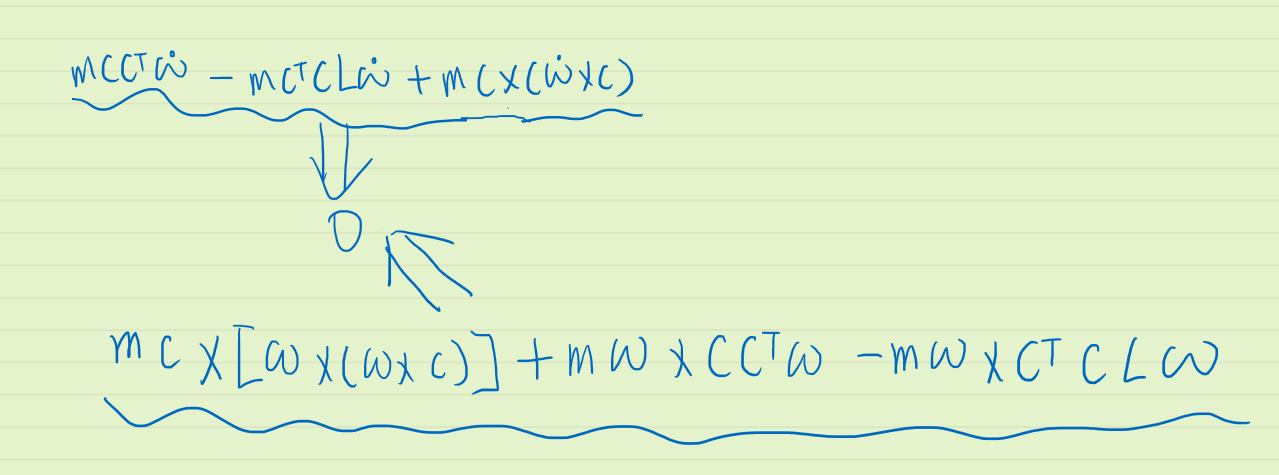验算：
clear,clc;
syms px py pz wx wy wz real;
c = [px;py;pz]
w = [wx;wy;wz]

temp1 = c*c'*w-c'*c*diag([1 1 1])*w+cross(c,cross(w,c))
simplify(temp1)

temp2 = cross(c,cross(w,cross(w,c))) + cross(w,c*c'*w)-cross(w,c'*c*diag([1 1 1])*w)
simplify(temp2)


展开全文• 翻译过程稍有删减  前面已经介绍了最基本的向量...在实际中，向量运算往往是这两种基本运算的复合，这就需要一些运算规则向量绝大部分的运算规则与标量对应的运算规则一致。  第一条规则是：向量相加满足交

原文链接：http://thejuniverse.org/PUBLIC/LinearAlgebra/LOLA/geomVect/calc.html
翻译过程稍有删减
前面已经介绍了最基本的向量相加及向量数乘。在实际中，向量的运算往往是这两种基本运算的复合，这就需要一些运算的规则。向量绝大部分的运算规则与标量对应的运算规则一致。
第一条规则是：向量相加满足交换律。用更数学化的语言可表述为：对任意向量u 和 v，有如下等式成立： u + v = v + u.
这点很好理解，下图为采用平行四边形法则的向量相加几何示意图，无论是u 和加v，还是  v加u，结果都是以u和v两个向量为边构成的平行四边形的对角线。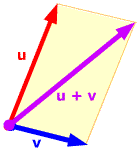若有三个或者三个以上的向量相加，结果又如何呢？三角形法则告诉我们，将这些向量的起点和终点相连，则以第一个向量的起点为起点，以最后一个向量的终点为终点，构成的这个向量即为和向量，如下图所示。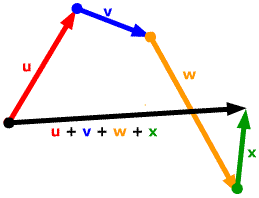上述规则无疑是正确的。但从技术上来讲，向量相加的法则仅定义了两个向量相加。因此，我们必须确保上述法则在只有两个向量相加时也是正确的。因此，我们还需要如下的规则：即向量相加满足结合律。用更数学化的语言可表述为：对任意向量u 、v和w，有如下等式成立：  (u + v)+ w = u + (v + w).
下图给出了向量加法结合律的几何图示。上面是等式左边的相加结果，下面是等式右边的相加结果。可以看出，结果都是黑色的向量。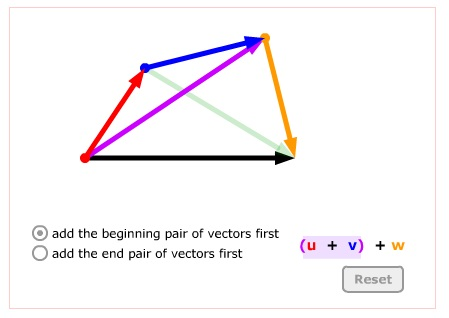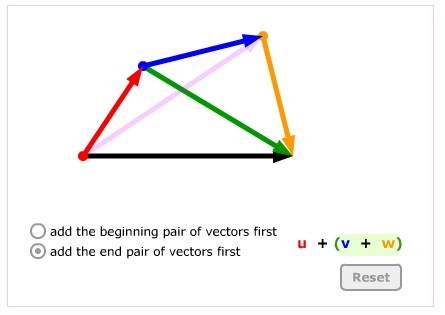利用交换律和结合律这两个规则，我们就可以解决任意多个向量以任意次序相加的问题。
前面我们仅给出了向量相加运算法则在二维空间的几何示意图。下图给出了三个向量在三维空间相加的几何示意图。图中三个向量处于不同的平面，我们可以先将他们的起点放置在一起，然后先利用平行四边形法则计算u + v，然后再利用三角形法则计算(u + v)+ w。由图可以看出，和向量实际上是由u、 v、w三个向量构成的平行六面体的对角线。因此，在三维空间，向量相加的法则也称为平行六面体法则，这是二维空间到三维空间的自然推广。在数学抽象上，这样的法则还可以推广到三维以上的空间，只是无法给出具体的几何示意图。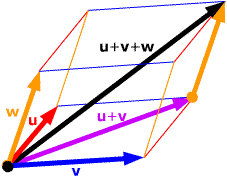上面我们讨论了多个向量相加的问题。对数乘运算，假定现在有两个数c 和 d，都要和向量v进行数乘运算，那么运算的次序有关系吗？可以证明，数乘运算时，先用那个数进行数乘并不影响最终的结果，即有：c(dv) =d(cv) = (cd)v.
如果c 和 d均为正数，那么很明显，上述三种运算所得的结果相同，并且仍然是一个向量，其方向与v相同，其长度为v的长度乘以cd。如果c 和 d有一个为负数或者两者均为负数，上述三种运算就必须要考虑方向的变反的情况，但注意到，在三种运算中，向量方向变反的次数是一样的，因此，三种运算所得的结果仍然相同。这即说明上述数乘满足交换律的运算规则是有效的。
我们再考虑更复杂一些的情况，几个向量之间的运算不仅有加法，还有数乘运算，这时就需要分配率。这里，分两种情况来讨论。
第一种情况是两个数相加后再与向量相乘。此时的运算规则如下：两个数相加之后再与向量相乘，等于两个数分别与向量相乘后再相加。用数学公式可表示为：对于任意的数c，d及任意的向量v，有：
(c + d)v =cv +dv.
如果c 和 d均为正数，如下图所示，那么从图很容易看出，上述等式两边的运算结果相同，结果均为向量，其方向与v相同，长度为v的长度乘以(c +d)。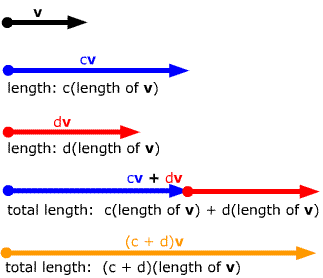如果c 和 d有一个为负数，或者均为负数，上述分配率规则同样有效，但所得向量的方向与(c+d)的取值有关，可能与v相同，也可能与v相反。
再来考虑另外一种情况，一个数与两个向量相加的结果相乘，此时的运算规则如下：一个数与两个向量相加的结果相乘，等于这个数分别与这两个向量单独相乘，然后再相加。用数学公式可表示为：对于任意的数c，d及任意的向量v，有：
c(u + v) = cu + cv.
对这条规则的理解与第一种情况的完全类似，下图给出了一个这种情况下的几何示意图。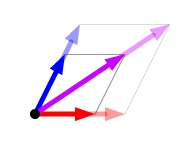综合这两种情况，用更口语化的方式，分配率可统一表述为：相加之后的相乘与相乘之后的相加相等。

展开全文• 运算法则：例如3d零向量表示为：[0,0,0] 几何解释：没有位移 2.负向量 运算法则： 每个分量都变负 数学表达： 几何解释： 向量变负，将得到一个和原来向量大小相等，方向相反的向量。 3.向量的大小（长度和模） ...


1.零向量
加性单位元：满足y+x=y
n维向量集合的加性单位元就是n维零向量
运算法则：例如3d零向量表示为：[0,0,0]
几何解释：没有位移
2.负向量
运算法则：
每个分量都变负
数学表达：几何解释：
向量变负，将得到一个和原来向量大小相等，方向相反的向量。3.向量的大小（长度和模）
运算法则：
n维向量大小计算公式为几何解释：
2d中任意向量v能构造一个以v为斜边的直角三角形如下图所示4.标量与向量乘法
运算法则：几何解释：
效果是以因子|k|缩放向量的长度，例如想让向量长度增加倍，应使向量乘以2标准化向量
运算法则：
向量除以它的大小（模）即可。几何解释：向量的加法和减法
运算法则：
加法减法解释为加负向量几何解释：
向量a+b解释为：使a的头连接b的尾，接着从a的尾向b的头画一个向量。这就是向量加法的“三角形法则”三角形法扩展到多个向量一个点到另一个点的向量
计算一个点到另一个点的位移是一种非常普遍的要示，可以使用三角形法则和向量减法来解释这个问题。距离公式：
说明：等于一个点到另一个点的向量的长度。
运算法则：
先求两点构成的向量d再计算d的模
||d||
向量点乘
运算法则：几何解释：
点乘结果描述了两个向量的“相似”程序，点乘结果越大，两向量越相近。点乘等于向量大小与向量加角的cos值的积解得：如果a,b是单位向量就可能避免上述公式中的余法运算如果不需要夹角的确切值只需要a和b的夹角类型，可以取用点乘结果的符号，如下图所示向量投影我们用点乘计算投影，下图给出和几何解释当然，如果n是单位向量，除法就不必要了向量叉乘
运算法则：几何解释：
叉乘得到的向量垂直与原来两个向量图中，向量a和b在一个平面中。向量a * b 指向该平面的正上方，垂直于a和b出处：
http://www.cnblogs.com/zhangdongsheng/

作者：
张东升


展开全文• 定比分点公式（向量P1P=λ•向量PP2）:设P1、P2是直线L上的两点，P是L上不同于P1、P2的任意一点。则存在一个实数 λ，使 向量P1P=λ•向量PP2，λ叫做点P分有向线段P1P2所成的比。 若P1（x1,y1)，P2(x2,y2)，P(x,y)...

定比分点公式（向量P1P=λ•向量PP2） :设P1、P2是直线L上的两点，P是L上不同于P1、P2的任意一点。则存在一个实数 λ，使 向量P1P=λ•向量PP2，λ叫做点P分有向线段P1P2所成的比。   若P1（x1,y1)，P2(x2,y2)，P(x,y)，则有 OP=(OP1+λOP2)(1+λ)；（定比分点向量公式）   x=(x1+λx2)/(1+λ),   y=(y1+λy2)/(1+λ)。（定比分点坐标公式）    三点共线定理 :若OC=λOA +μOB ,且λ+μ=1 ,则A、B、C三点共线 。  三角形重心判断式 :在△ABC中，若GA +GB +GC=O,则G为△ABC的重心 。向量共线的重要条件 ： 若b≠0，则a//b的重要条件是存在唯一实数λ，使a=λb。 a//b的重要条件是 xy'-x'y=0。   零向量0平行于任何向量。 向量垂直的充要条件   a⊥b的充要条件是 a•b=0。   a⊥b的充要条件是 xx'+yy'=0。   零向量0垂直于任何向量.   1、向量的加法   向量的加法满足平行四边形法则和三角形法则。   AB+BC=AC。   a+b=(x+x'，y+y')。   a+0=0+a=a。   向量加法的运算律：   交换律：a+b=b+a；   结合律：(a+b)+c=a+(b+c)。
2、向量的减法   如果a、b是互为相反的向量，那么a=-b，b=-a，a+b=0. 0的反向量为0   AB-AC=CB. 即“共同起点，指向被减”   a=(x,y) b=(x',y') 则 a-b=(x-x',y-y').    3、向量的数量积   定义：已知两个非零向量a,b。作OA=a,OB=b,则角AOB称作向量a和向量b的夹角，记作〈a,b〉并规定0≤〈a,b〉≤π   定义：两个向量的数量积（内积）是一个数量，记作a•b。若a、b不共线，则a•b=|a|•|b|•cos〈a，b〉；若a、b共线，则a•b=+-∣a∣∣b∣。   向量的数量积的坐标表示：a•b=x•x'+y•y'。   向量的数量积的运算律   a•b=b•a（交换律）；   (λa)•b=λ(a•b)(关于数乘法的结合律)；   （a+b)•c=a•c+b•c（分配律）；   向量的数量积的性质   a•a=|a|的平方。   a⊥b 〈=〉a•b=0。   |a•b|≤|a|•|b|。   向量的数量积与实数运算的主要不同点   1、向量的数量积不满足结合律，即：(a•b)•c≠a•(b•c)；例如：(a•b)^2≠a^2•b^2。   2、向量的数量积不满足消去律，即：由 a•b=a•c (a≠0)，推不出 b=c。   3、|a•b|≠|a|•|b|   4、由 |a|=|b| ，推不出 a=b或a=-b。 4、数乘向量   实数λ和向量a的乘积是一个向量，记作λa，且∣λa∣=∣λ∣•∣a∣。   当λ＞0时，λa与a同方向；   当λ＜0时，λa与a反方向；   当λ=0时，λa=0，方向任意。   当a=0时，对于任意实数λ，都有λa=0。   注：按定义知，如果λa=0，那么λ=0或a=0。   实数λ叫做向量a的系数，乘数向量λa的几何意义就是将表示向量a的有向线段伸长或压缩。   当∣λ∣＞1时，表示向量a的有向线段在原方向（λ＞0）或反方向（λ＜0）上伸长为原来的∣λ∣倍；   当∣λ∣＜1时，表示向量a的有向线段在原方向（λ＞0）或反方向（λ＜0）上缩短为原来的∣λ∣倍。   数与向量的乘法满足下面的运算律   结合律：(λa)•b=λ(a•b)=(a•λb)。   向量对于数的分配律（第一分配律）：(λ+μ)a=λa+μa.   数对于向量的分配律（第二分配律）：λ(a+b)=λa+λb.   数乘向量的消去律：① 如果实数λ≠0且λa=λb，那么a=b。② 如果a≠0且λa=μa，那么λ=μ。
5、向量的向量积 、外积  定义：两个向量a和b的向量积（外积、叉积）是一个向量，记作a×b。a×b的方向是：垂直于a和b，且a、b和a×b按这个次序构成右手系。若a、b共线，则a×b=0。 如果向量是二维的 ：( a =   (ax, by) , b = (bx, by)   )，那么a x b = ax * by - ay * bx = |a| * |b| * sin<a, b>如果向量是三维的 ：a = (ax, ay, az), b = (bx, by, bz); 则 a x b = (ay * bz - by * az, az * bx - ax * bz, ax * by - ay * bx);符合三维矩阵算法  向量的向量积性质：   ∣a×b∣是以a和b为边的平行四边形面积。   a×a=0。   a‖b〈=〉a×b=0。   向量的向量积运算律   a×b=-b×a；   （λa）×b=λ（a×b）=a×（λb）；   （a+b）×c=a×c+b×c.   注：向量没有除法，“向量AB/向量CD”是没有意义的。    向量的三角形不等式   1、∣∣a∣-∣b∣∣≤∣a+b∣≤∣a∣+∣b∣；   ① 当且仅当a、b反向时，左边取等号；   ② 当且仅当a、b同向时，右边取等号。   2、∣∣a∣-∣b∣∣≤∣a-b∣≤∣a∣+∣b∣。   ① 当且仅当a、b同向时，左边取等号；   ② 当且仅当a、b反向时，右边取等号。

转载于:https://www.cnblogs.com/wang-jin-fu/p/8279725.html
展开全文• 四元数与三维向量相乘运算法则 参考网站： https://www.cnblogs.com/jeason1997/p/9822353.html 四元数和向量相乘可以表示这个向量按照这个四元数进行旋转之后得到的新的向量。 例如：向量 c(0,0,10) 绕着Y轴旋转90...
• 利用克莱姆法则实现三维空间中平面的法向量计算 取平面上三点分别为： P1(x1y1z1) P2(x2y2z2) P3(x3y3z3)求解设法向量(dxdydz)
• 向量加法满足平行四边形法则和三角形法则向量加法的运算律有交换律a+b=b+a；结合律(a+b)+c=a+(b+c)。向量的减法：如果a、b是互为相反的向量，a+b=0。向量的加减法向量加法的运算律交换律：a+b=b+a；结合律：(a+b)+...
• 理解向量运算 http://www.ceeger.com/Manual/UnderstandingVectorArithmetic.html 射影长度 立体几何中怎样用向量法求直线在一个面上的射影长度 答 首先说，问题应该是线段在一个面上的射影长度...
• 高中数学 第二章 平面向量运算法则复习导学案 新人教版必修4
• Unity3D 中常用的向量运算 那么接下来来看一看在游戏开发过程中常用的一些向量运算 向量的叉积 / 叉乘 叉积的定义（cross product） 叉积的计算结果依然是一个向量，叉积只能用于3D向量的运算，2D向量没有叉积 。 ...unity 算法
• 而数组运算是 MATLAB 软件所定义 的规则 ,其目的是为了数据管理方面 ,操作简单 ,指令形式自然和执行计算有效 .所以 ,在使用 MATLAB 时 ,特别要明确搞清数组运算和矩阵运算的区别 .表 4.1.1 列出了两种运
• ## 数学基础 —— 向量运算（叉乘）

万次阅读 多人点赞 2016-08-05 17:17:57
向量的叉乘，求通知垂直两个向量向量，即c垂直于a，同时c垂直于b（a与c的夹角为90°，b与cc的夹角为90°） c = a×b = （a.y*b.z-b.y*a.z , b.x*a.z-a.x*b.z , a.x*b.y-b.x*a.y） 以上图为例a(1,0,0),b(0,1,0),...
• 第1节：零向量 1.零向量的概念 　对于任意向量x，都有x+y=x，则x被称为零向量。例如，3D零向量为[0 0 0]。零向量非常特殊，因为它是唯一...2.负向量运算法则 　 　将此法则应用到2D，3D，4D中，则 　-[x y...
• 向量加法的定义及运算法则PPT学习教案.pptx
• 向量运算向量 要得到任意维向量的负向量，只需要简单的将向量每个分量都变负即可。数学表达式： −[ab...x]=[−a−b...−x]\begin{gathered} -\begin{bmatrix}a\\b\\...\\x\end{bmatrix} = \begin{bmatrix}-a\...线性代数...